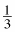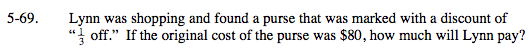### Home > MC2 > Chapter 5 > Lesson 5.2.3 > Problem5-69

5-69.

Lynn was shopping and found a purse that was marked with a discount of "off." If the original cost of the purse was $80, how much will Lynn pay? Homework Help ✎$\text{Find }\frac{1}{3} \text{ of \80 to figure out how much the discount is.}$ Multiply the two values together: $\left(\frac{1}{3}\right)(80)=26.67$ Lynn's discount is$26.67. How much will she have to pay after the discount?